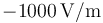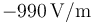# Discussion Forum

## Using the minimum/maximum value in the plot range

I have a surface plot of the z-component of the electric field in a simulation I ran (though I suspect that the question is independent of the value I am plotting) and I want to restrict the color range to the lowest (or highest) one percent of field values. So, if the minimum field in the cut plane is, I want to set the color range minimum toand the maximum to.

However, when I change some parameter and recalculate the simulation, the minimum field value will change, so I want to enter this in a more general fashion. I imagine writing something like `min(es.Ez)` and `0.99 * min(es.Ez)`, but obviously that is not the right syntax.

How can I do this?

6 Replies Last Post Jan 20, 2022, 10:40 a.m. EST

Posted: 4 months ago

I suggest that you add a Filter subnode to the plot and use the following logical expression in the Filter:

(es.Ez >= -1000[V/m]) && (es.Ez <= -990[V/m])

Posted: 4 months ago

Thanks for the answer, though this doesn't really address my problem. Adjusting the color range does what I want to do, where I'm having problems is automatically calculating the limits (-1000[V/m] and -990[V/m] in the example). If I change a parameter and recalculate the solution so the minimum field value is now -1200[V/m], I want the limits to automatically adjust to -1200[V/m] and -1188[V/m].

Posted: 4 months ago

Try the following: * Component > Definitions: Add a Nonlocal Couplings > Maximum. Select the domains where you want to compute the maximum. * Add a Nonlocal Couplings > Minimum in the same way. * Study > Update Solution: This will add the maximum and minimum couplings defined above. * You can now use an operator syntax in the Filter to evaluate the maximum and minimum of an expression over the domains selected in the nonlocal couplings. E.g.,

es.Ez >= 0.99maxop1(es.Ez)+0.01minop1(es.Ez)

Here maxop1 and minop1 are the Operator names in the nonlocal couplings.

It is not possible to use these operators in the color range.

Posted: 4 months ago

Hmm, ok, too bad that I can't use these in the color range.

Is it possible to instead use parameters to set these limits? (I have quite a few plots that should use the same limits, so this would be the next best thing.) I managed doing it with model parameters but that is quite cumbersome as I have to first run the simulation, then set the parameters and then update the simulation again to make the new parameter values available for plotting. Adding the parameters to the Results node failed with an "unknown model parameter" error.

Posted: 4 months ago

You can use model parameters but not result parameters in the limit in the color range.

To simplify management of limits you can add variables to Component > Definitions > Variables that contain the limits. That way minop and maxop can be confined to one place.